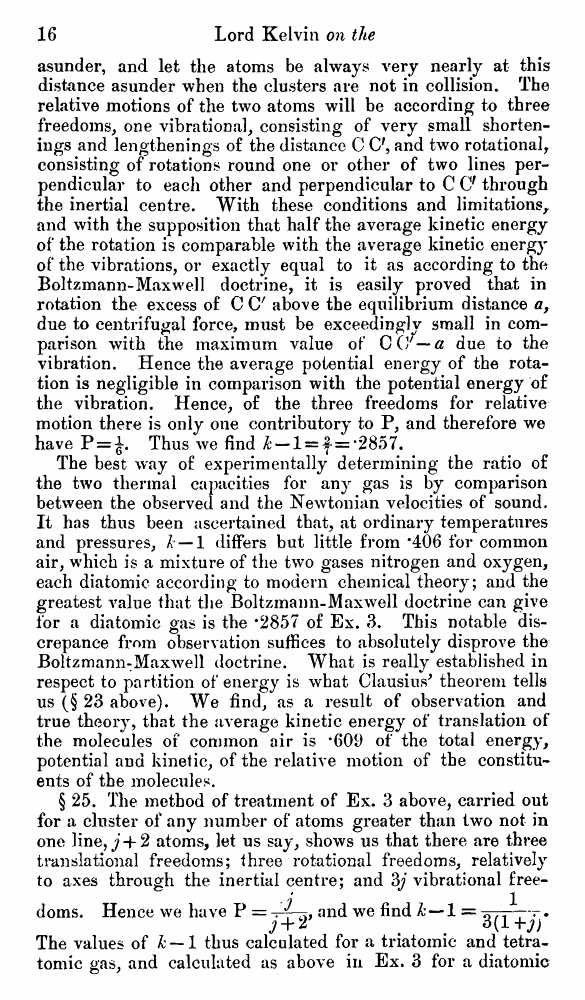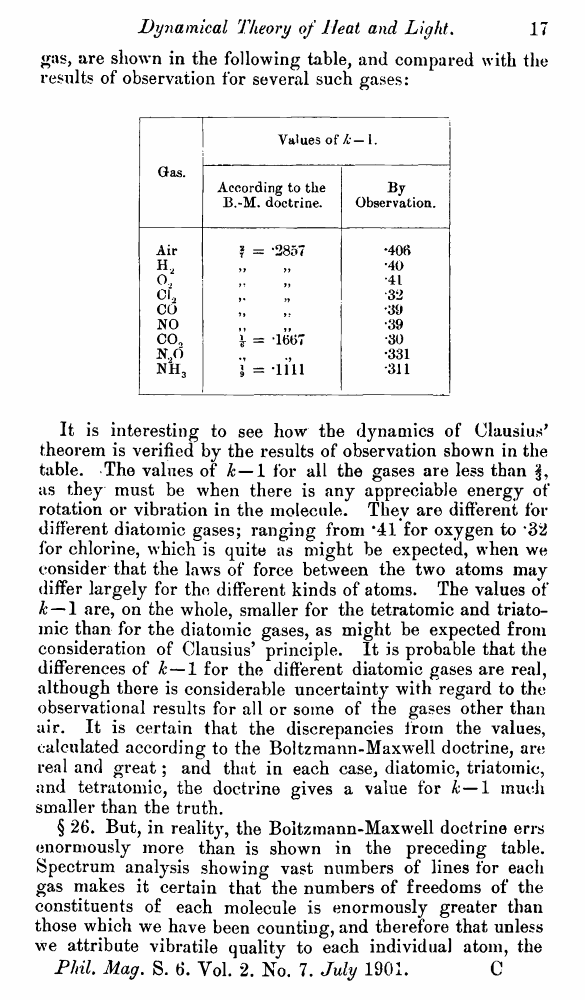﻿ ﻿ Lord Kelvin. Nineteenth-Century Clouds over the Dynamical Theory of Heat and Light. // Phil. Mag. S. 6. Vol. 2. No. 7. July 1901.

# Lord Kelvin. Nineteenth-Century Clouds over the Dynamical Theory of Heat and Light. // Phil. Mag. S. 6. Vol. 2. No. 7. July 1901.

В начало   Другие форматы   <<<     Страница 16   >>>1  2  3  4  5  6  7  8  9  10  11  12  13  14  15  16 17  18  19  20  21  22  23  24  25  26  27  28  29  30  31  32  33  34  35  36  37  38  39  40

asunder, and let the atoms be always very nearly at this distance asunder when the clusters are not in collision. The relative motions of the two atoms will be according to three freedoms, one vibrational, consisting of very small shortenings and lengthenings of the distance C C', and two rotational, consisting of rotations round one or other of two lines perpendicular to each other and perpendicular to C O through the inertial centre. With these conditions and limitations, and with the supposition that half the average kinetic energy of the rotation is comparable with the average kinetic energy of the vibrations, or exactly equal to it as according to the Boltzmann-Maxwell doctrine, it is easily proved that in rotation the excess of C C' above the equilibrium distance a, due to centrifugal force, must be exceedingly small in comparison with the maximum value of CO7 — a due to the vibration. Hence the average potential energy of the rotation is negligible in comparison with the potential energy of the vibration. Hence, of the three freedoms for relative motion there is only one contributory to P, and therefore we have P = ^. Thus we find & —l = |- = *2857.

The best way of experimentally determining the ratio of the two thermal capacities for any gas is by comparison between the observed and the Newtonian velocities of sound. It has thus been ascertained that, at ordinary temperatures and pressures, h — 1 differs but little from *406 for common air, which is a mixture of the two gases nitrogen and oxygen, each diatomic according to modern chemical theory; and the greatest value that the Boltzmann-Maxwell doctrine can give for a diatomic gas is the *2857 of Ex. 3. This notable discrepance from observation suffices to absolutely disprove the Boltzmann:Maxwell doctrine. What is really established in respect to partition of energy is what Clausius' theorem tells us (§ 23 above). We find, as a result of observation and true theory, that the average kinetic energy of translation of the molecules of common air is *609 of the total energy, potential and kinetic, of the relative motion of the constituents of the molecules.

§ 25. The method of treatment of Ex. 3 above, carried out for a cluster of any number of atoms greater than two not in one line, j-1-2 atoms, let us say, shows us that there are three translational freedoms; three rotational freedoms, relatively to axes through the inertial centre; and 3j vibrational free-

? 1 doms. Hence we have P = —and we find & — 1 = 0.---r•

3 + 1 . . 3(1 +j)

The values of k — 1 thus calculated for a triatomic and tetra-tomic gas, and calculated as above in Ex. 3 for a diatomic

gas, are shown in the following table, and compared with the results of observation for several such gases:

 Gas. Values of h — 1. According to the B.-M. doctrine. B j Observation. Air ? == *2857 *406 H, •40 0, •41 CL 32 CO •a >• '39 NO •39 C0o \ = -16C7 ■30 N,0 •331 nh3 1 = li’ll *311

It is interesting to see how the dynamics of Clausius' theorem is verified by the results of observation shown in the table. The values of k — 1 for all the gases are less than as they must be when there is any appreciable energy of rotation or vibration in the molecule. They are different for different diatomic gases; ranging from *41 for oxygen to *32 for chlorine, which is quite as might be expected, when we consider that the laws of force between the two atoms may differ largely for the different kinds of atoms. The values of k — 1 are, on the whole, smaller for the tetratomic and triato-mic than for the diatomic gases, as might be expected from consideration of Clausius’ principle. It is probable that the differences of k—1 for the different diatomic gases are real, although there is considerable uncertainty with regard to the observational results for all or some of the gases other than air. It is certain that the discrepancies from the values, calculated according to the Boltzmann-Maxwell doctrine, are real and great; and that in each case, diatomic, triatomic, and tetratomic, the doctrine gives a value for k—1 much smaller than the truth.

§ 26. But, in reality, the Boltzmann-Maxwell doctrine errs enormously more than is shown in the preceding table. Spectrum analysis showing vast numbers of lines for each gas makes it certain that the numbers of freedoms of the constituents of each molecule is enormously greater than those which we have been counting, and therefore that unless we attribute vibratile quality to each individual atom, the Phil. Mag. S. 6. Vol. 2. No. 7. July 1901. C